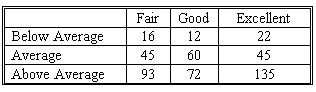### CFA Practice Question

There are 410 practice questions for this study session.

### CFA Practice Question

Each salesperson in a large department store chain is rated either below average, average, or above average with respect to sales ability. Each salesperson is also rated either fair, good, or excellent with respect to potential for advancement. These traits for 500 salespeople were cross classified into the following table.What is the probability that a sales person selected at random will have below-average sales ability and fair potential for advancement?
A. 0.16
B. 0.032
C. 0.10
Explanation: Prob. of selecting a below-average person: (16 + 12 + 22)/500 = 1/10

Prob. of selecting fair potential amongst those in the below-average group: 16/50 = 8/25

1/10*8/25 = 4/125